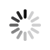#### Welcome!

×Forgot password?
Go back to Challenges list

Challenge

## Multi Operation Calculator

In this challenge you need to build a calculator that can perform multiple operation like add, subtract, multiply and divide at the same time.The operation symbols are +, -, * and /. There will be no parenthesis on the mathematical expressions but remember that * and / operators have precedence over + and -.

###### Input Format
Each test case contains the N numbers and N-1 symbols interspersed for the multiple mathematical operations. All of them are separated by a blank space and all numbers are integers.

###### Output Format
For each test case output the operation result.

###### Sample Inputs
```15 + 2 - 4 + 2 -4 / 2 + 2 ```

###### Sample Outputs
```15 0```

`512M`